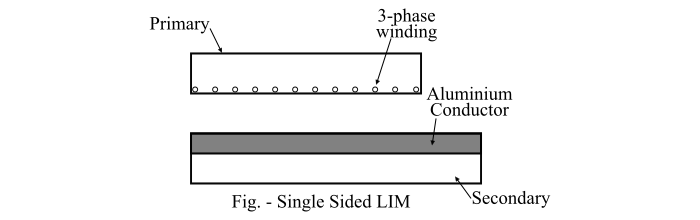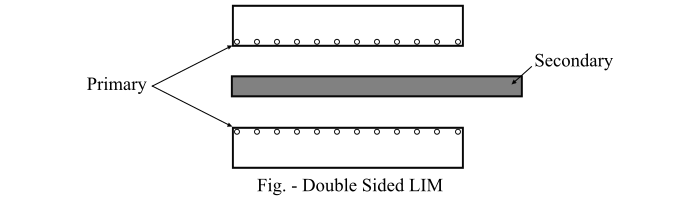# Linear Induction Motor: Design, Performance, and Applications

A linear induction motor (LIM) is an induction motor that converts electrical energy input into linear or translational motion instead of rotational motion as in the case of a conventional induction motor.

In a linear induction motor, the stator and the rotor are known as primary and secondary respectively. The secondary of the linear induction motor consists of a flat aluminium conductor with a ferromagnetic core. If the primary of the linear induction motor is connected to a three-phase supply, a traveling flux wave is produced which travels along the length of the primary.

Due to the relative motion between the traveling flux wave and the aluminium conductor, a current is induced in the aluminium conductor. This induced current also produces a magnetic flux wave which interacts with the traveling flux wave of the primary to produce a linear force F.

If the secondary of the linear induction motor is fixed and the primary is free to move, then the force will move the primary in the direction of the traveling flux wave.

## Design of Linear Induction Motor

There are two basic designs are used for construction of linear induction motors, which are given below −

If the linear induction motor has primary on one side of the secondary, then it is known as single-sided linear induction motor (SLIM).When it has primary on both the sides of the secondary, then it is known as double-sided linear induction motor (DLIM).## Performance of Linear Induction Motor

The linear synchronous speed vs of the travelling flux wave produced by the primary of the linear induction motor is given by

$$\mathrm{𝑣_{𝑠} = 2𝑓 × (Pole\:pitch) … (1)}$$

Where, f is the frequency of the supply voltage in Hz.

As the LIM is an asynchronous or induction motor, thus the speed of the secondary is less the synchronous speed and the difference between the two speed is known as slip. The slip of the linear induction motor is given by,

$$\mathrm{𝑠 =\frac{𝑣_{𝑠} − 𝑣_{𝑟}}{𝑣_{𝑠}}… (2)}$$

Therefore, the speed of the secondary vr in the linear induction motor is given by,

$$\mathrm{𝑣_{𝑟} = (1 − 𝑠)𝑣_{𝑠} … (3)}$$

The linear force or thrust of the linear induction motor is given by,

$$\mathrm{𝐹 =\frac{Air\:gap\:power\:(𝑃_{𝑔})}{Linear\:synchronous \:speed\:(𝑣_{𝑠})}… (4)}$$

## Drawbacks of Linear Induction Motor

A linear induction motor (LIM) has the following disadvantages −

• The linear induction motor requires a larger air-gap as compared to a conventional rotary induction motor.

• The magnetising current drawn by a linear induction motor is larger than that of a rotary induction motor of same rating.

• The efficiency and the power factor of a linear induction motor are lower than that of a conventional induction motor of the same rating.

## Applications of Linear Induction Motor

The applications of the linear induction motor are given below −

• The primary application of linear induction motor is in transportation, including electric traction where the primary is mounted on the vehicle and the secondary laid along the track.

• Linear induction motors are used in cranes for material handling.

• Also used for pumping of liquid metal.

• Used as actuators for door movement.

• Linear induction motors are used in high-voltage circuit breakers.

• Linear induction motors are also used in accelerators for rigs for testing vehicle performance under impact conditions.